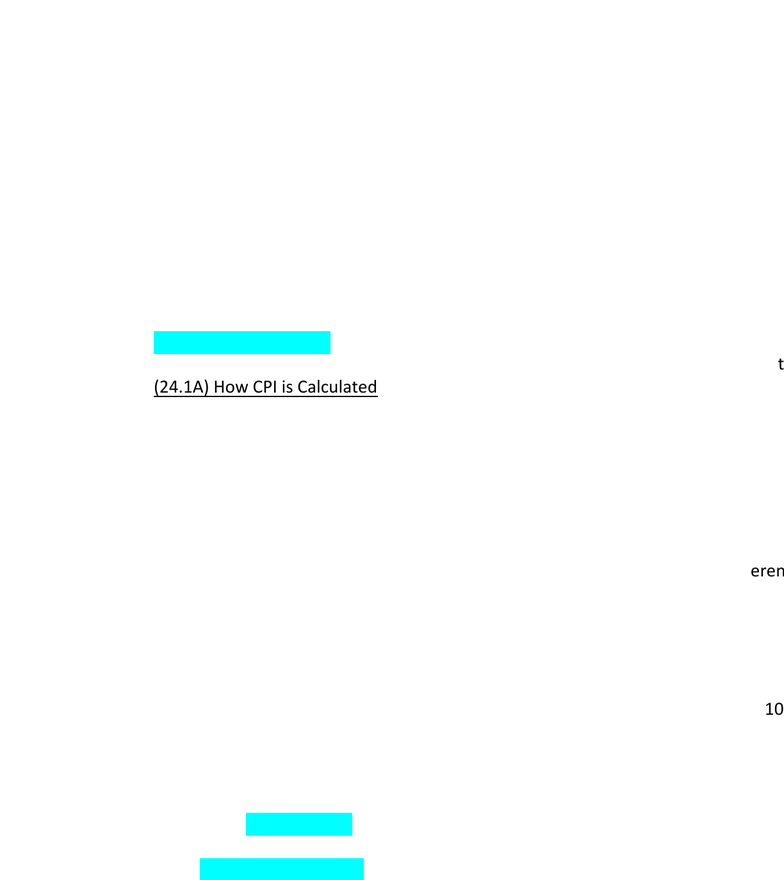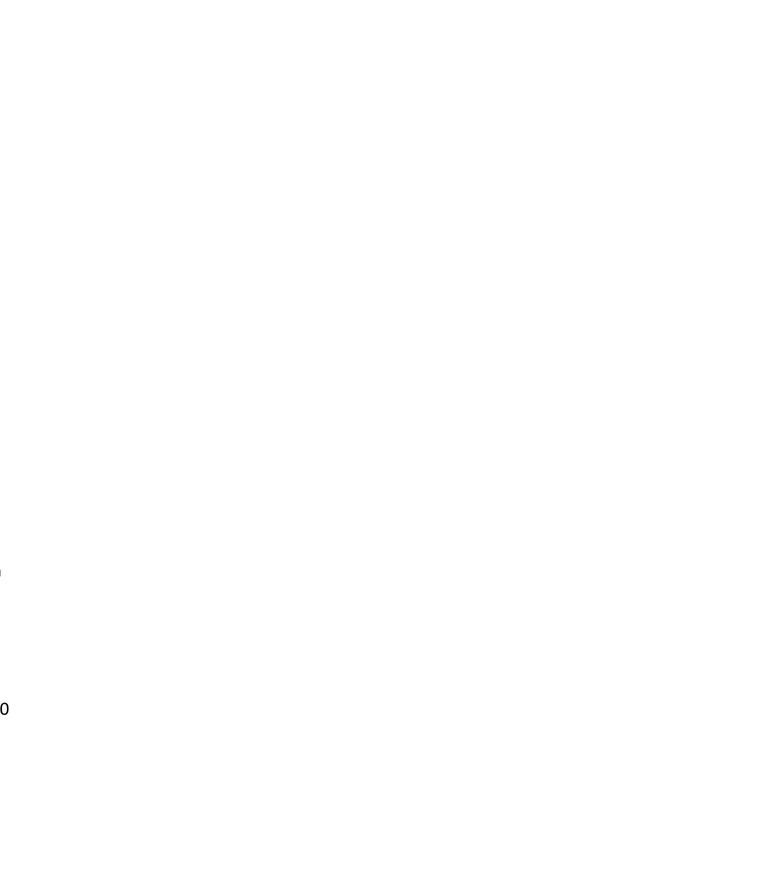Textbook Notes (280,000)
US (110,000)
UCLA (2,000)
ECON (100)
ECON 2 (10)
Chapter 24

# ECON 2 Chapter Notes - Chapter 24: Real Interest Rate, Interest Rate, Nominal Interest Rate

Department
Economics
Course Code
ECON 2
Professor
Keskinel, M.
Chapter
24

This preview shows pages 1-2. to view the full 6 pages of the document.Introduction
Consumer price index
Used to monitor changes in cost of living over time
i.
Rises --> spend more money to maintain same standard of living
Inflation
1)
ii.
a.
Inflation rate
Percentage change in price level form previous period
i.
b.
(24.1) Consumer Price Index
Consumer price index:
Measure of the overall cost of the good and services bought by a typical custo
i.
a.
(24.1A) How CPI is Calculated
Uses data on the prices of thousands of G/S
i.
Determine which prices are most important to typical consumer
1)
Weigh the more important cost more heavily
2)
ii.
Step 2: Find the prices
Find the prices of each of the G/S in the basket at each point in time
1)
iii.
Step 3: Compute the basket's cost
Use data on prices to calculate the cost of the basket of G/S at different
1)
Only prices in calculation change
a)
2)
iv.
Step 4: Choose a base year and compute the index
Designate one year as the base year
1)
Choice is arbitrary
2)
CPI = (\$ of basket of G/S in current year / \$ of basket in base year) x 100
3)
CPI is always 100 in base year
4)
v.
Step 5: Compute the inflation rate
Use CPI to calculate inflation rate
1)
Inflation rate in year 2 = [(CPI in yr. 2 - CPI in yr. 1)/CPI in yr. 1] x 100
2)
Inflation rate:
% change in price index from preceding year
a)
3)
vi.
Producer price index:
vii.
b.
Chapter 24: Measuring the Cost of Living
Saturday, April 29, 2017
15:49

Only pages 1-2 are available for preview. Some parts have been intentionally blurred.ustomer
ent times
100